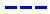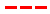Magic Kingdom attraction wait times for December 31, 2014.

•The Average Wait Time We PredictedThe Average Wait Time We Saw
•The Average Wait Time We PredictedThe Average Wait Time We Saw
•The Average Wait Time We PredictedThe Average Wait Time We Saw
•The Average Wait Time We PredictedThe Average Wait Time We Saw
•The Average Wait Time We PredictedThe Average Wait Time We Saw
•The Average Wait Time We PredictedThe Average Wait Time We Saw
•The Average Wait Time We PredictedThe Average Wait Time We Saw
•The Average Wait Time We PredictedThe Average Wait Time We Saw
•The Average Wait Time We PredictedThe Average Wait Time We Saw
•The Average Wait Time We PredictedThe Average Wait Time We Saw
•The Average Wait Time We PredictedThe Average Wait Time We Saw
•The Average Wait Time We PredictedThe Average Wait Time We Saw
•The Average Wait Time We PredictedThe Average Wait Time We Saw
•The Average Wait Time We PredictedThe Average Wait Time We Saw
•The Average Wait Time We PredictedThe Average Wait Time We Saw
•The Average Wait Time We PredictedThe Average Wait Time We Saw
•The Average Wait Time We PredictedThe Average Wait Time We Saw
•The Average Wait Time We PredictedThe Average Wait Time We Saw
•The Average Wait Time We PredictedThe Average Wait Time We Saw
•The Average Wait Time We PredictedThe Average Wait Time We Saw
•The Average Wait Time We PredictedThe Average Wait Time We Saw
•The Average Wait Time We PredictedThe Average Wait Time We Saw
•The Average Wait Time We PredictedThe Average Wait Time We Saw
•The Average Wait Time We PredictedThe Average Wait Time We Saw
•The Average Wait Time We PredictedThe Average Wait Time We Saw
•The Average Wait Time We PredictedThe Average Wait Time We Saw
•The Average Wait Time We PredictedThe Average Wait Time We Saw
•The Average Wait Time We PredictedThe Average Wait Time We Saw
•The Average Wait Time We PredictedThe Average Wait Time We Saw